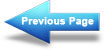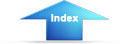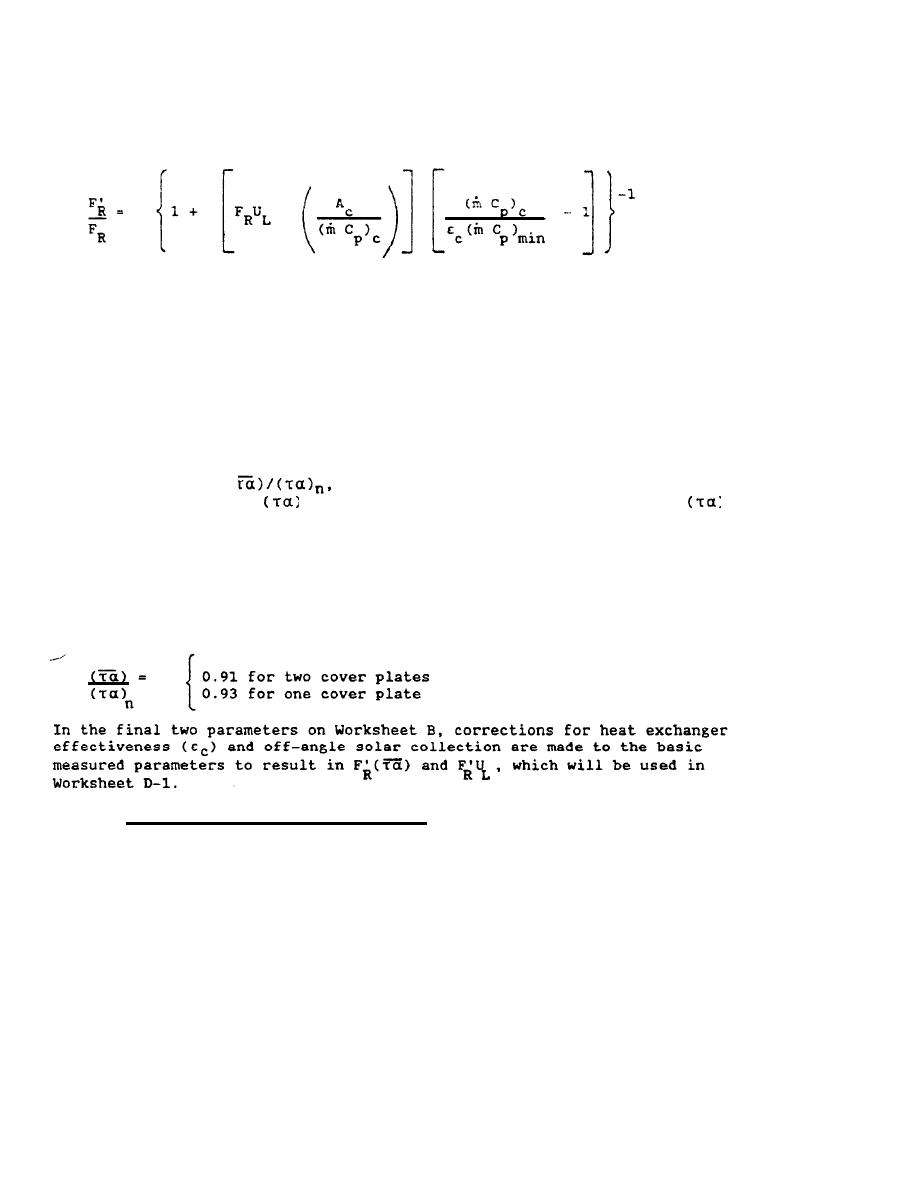Custom SearchDesign: Solar Heating of Buildings and Domestic Hot WaterMIL-HDBK-1003/13A
The term (mCp)c/(mCp)min is the ratio of the heat capacity flow rates
in the collector-tank heat exchanger.  The subscript "c" refers to the
collector flow stream; the subscript "min" refers to whichever of the two
flowrates has the lesser value.
The ratio F'R/FR, line 6, Worksheet B, where F'R is the collector-tank
heat exchanger efficiency factor is calculated from the equation using the
factors previously developed.  If there is to be no heat exchanger, then this
ratio equals 1.0 for air and liquid systems.
Typically, if a heat exchanger is used with an effectiveness of 0.7 as assumed
above and if the collector flow (mCp)c, is about the same as the other
(storage) flow, (mCp)min, as is often the case, then the ratio F'R/FR
is about 0.95 to 0.97 for a liquid system.  This is not true for air systems
which must be calculated.  See U.S. Dept HUD (1977). pages A-26 and A-27 for
further calculations of this parameter.
The next factor is
line 7 Worksheet B, where the bar refers
to an average value of
and subscript "n" refers to the value of
taken with the sun normal to the collector.  This factor represents the
variations in transmittance and absorptance due to changes in the sun angle
during the day.  Solar collectors are tested near solar noon, and there is a
reduction in transmission of insolation at high angles of incidence which
occur in early morning or late afternoon.  This reduction (called incident
angle modifier) may be available from the manufacturer of the collector since
it is a parameter that is measured as part of normal ASHRAE 93-77 performance
test.  If it is not available it may be taken as a constant as follows:
3.3 Load calculations - Worksheet C-l.  Worksheet C-l is an aid to
calculating the space heating and DHW load for family housing.  For other
buildings use conventional methods of calculating load; computer programs are
available for this.  For existing buildings, heating load may be inferred from
fuel bills, if available, see Example 1 (Section 4.1); or the Btu/ft  2
degree-day (dd) method of Worksheet C-l may be used.  Table 3-1 gives
estimated Btu/ft2-dd heat loss rates for various structural types used in
family housing.  If net heat loss rate is based on amount of fuel used, load
is gross load and must be multiplied by furnace efficiency to get net heating
90Integrated Publishing, Inc. - A (SDVOSB) Service Disabled Veteran Owned Small Business